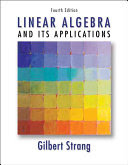### Linear Algebra

Data is displayed for academic year: 2023./2024.

#### Course Description

Matrix calculus, determinants, solving linear systems of equations. Vector analysis and analytic geometry of space. Vector spaces, linear operators, eigenvalues and eigenvectors, operator diagonalization.

#### Study Programmes

[FER3-EN] Computing - study
(1. semester)
[FER3-EN] Electrical Engineering and Information Technology - study
(1. semester)

#### Learning Outcomes

1. describe and apply linear algebra basic concepts and methods
2. demonstrate fundamental skills of matrix calculus and solving linear systems of equations
3. apply fundamental knowledge of vector analysis and space analytic geometry
4. demonstrate basic knowledge of vector spaces and linear operators
5. demonstrate an ability to express mathematical ideas and abstract thinking in linear algebra
6. demonstrate an ability to basic problem solving and reaching conclusions in linear algebra
7. use methods of linear algebra in engineering

#### Forms of Teaching

Lectures

Lectures are held in two cycles, 4 hours per week

Exercises

Exercises are held in two cycles, 4 hours per week

Partial e-learning

Homework is accessible on course web-page.

Continuous Assessment Exam
Homeworks 50 % 0 % 0 % 0 %
Attendance 50 % 0 % 0 % 0 %
Mid Term Exam: Written 0 % 50 % 0 %
Final Exam: Written 0 % 50 %
Exam: Written 0 % 100 %
##### Comment:

Regular attendance of lectures and doing homework are requirement for taking exam.

#### Week by Week Schedule

1. Matrices; Basic types; Operations with matrices; Inverse matrix; Regular and singular matrices
2. Determinants; Basic properties, computation of determinants; Laplace's rule, Elementary transformations, rank of a matrix, linear independence and rank; Characterization of regular matrices
3. Elementary transformations, rank of a matrix, linear independence and rank; Characterization of regular matrices, Characterization of regular matrices using determinant; Computation of the inverse matrix
4. Gaussian elimination method; Homogeneous and nonhomogeneous systems, Rank of a system and rank of extended matrix
5. Rank of a system and rank of extended matrix, Cramer's rule; Comparison of computational complexity of the Gauss and Cramer algorithms
6. Operations with vectors; Linear dependence and independence in V^2 and V^3; Bases in V^2 and V^3; Canonical base; Vectors in coordinate systems, Dot product; Norm, ortogonality; Vector and scalar projections of one vector on another
7. Cross product of two vectors and mixed product of three vectors, Radius-vector; Coordinates of the midpoint of a segment and of the barycenter of a triangle; Convex combinations of vectors; Convex hull; Convex set
8. Midterm exam
9. Equations of plane; Mutual position of two planes; Distance between a point and a plane
10. Line, parametric and canonical equations; Mutual position of a line and a plane
11. Vector spaces and their subspaces; Linear hull (span); The space R^n, Linear independence and dependence; Basis and dimension
12. Coordinate system; Basis change; Transition matrix, Inner product; Inner product space; Orthogonal basis; Fourier coefficient; Orthogonal projection; Normed vector space
13. Linear operators and their matrix representation; Basis change; Similar matrices, Examples of linear operators in V^2 and V^3; Linear functional; Hyperspace, half-space
14. Eigenvalues and eigenvectors; Eigenspaces; Characteristic polynomial; Hamilton-Cayley's theorem, Schur's theorem; Matrix functions; Spectral mapping theorem
15. Final exam

#### Literature

Neven Elezović (2016.), Linearna algebra, Element, Zagreb
N. Elezović, A. Aglić Aljinović (2006.), Linearna algebra: zbirka zadataka, Element, ZagrebGilbert Strang (2006.), Linear Algebra and its Applications, Brooks Cole
Damir Bakić (2008.), Linearna algebra, Školska knjiga, Zagreb
David S. Watkins (2002.), Fundamentals of Matrix Computations, Wiley

#### General

ID 209622
Winter semester
5 ECTS
L0 English Level
L2 e-Learning
60 Lectures
0 Seminar
15 Exercises
0 Laboratory exercises
0 Project laboratory